# Demand forecasting with the Temporal Fusion Transformer#

In this tutorial, we will train the TemporalFusionTransformer on a very small dataset to demonstrate that it even does a good job on only 20k samples. Generally speaking, it is a large model and will therefore perform much better with more data.

Our example is a demand forecast from the Stallion kaggle competition.

:

import os
import warnings

warnings.filterwarnings("ignore")  # avoid printing out absolute paths

os.chdir("../../..")

:

import copy
from pathlib import Path
import warnings

import lightning.pytorch as pl
from lightning.pytorch.callbacks import EarlyStopping, LearningRateMonitor
from lightning.pytorch.loggers import TensorBoardLogger
import numpy as np
import pandas as pd
import torch

from pytorch_forecasting import Baseline, TemporalFusionTransformer, TimeSeriesDataSet
from pytorch_forecasting.data import GroupNormalizer
from pytorch_forecasting.metrics import MAE, SMAPE, PoissonLoss, QuantileLoss
from pytorch_forecasting.models.temporal_fusion_transformer.tuning import optimize_hyperparameters


First, we need to transform our time series into a pandas dataframe where each row can be identified with a time step and a time series. Fortunately, most datasets are already in this format. For this tutorial, we will use the Stallion dataset from Kaggle describing sales of various beverages. Our task is to make a six-month forecast of the sold volume by stock keeping units (SKU), that is products, sold by an agency, that is a store. There are about 21 000 monthly historic sales records. In addition to historic sales we have information about the sales price, the location of the agency, special days such as holidays, and volume sold in the entire industry.

The dataset is already in the correct format but misses some important features. Most importantly, we need to add a time index that is incremented by one for each time step. Further, it is beneficial to add date features, which in this case means extracting the month from the date record.

:

from pytorch_forecasting.data.examples import get_stallion_data

data = get_stallion_data()

data["time_idx"] = data["date"].dt.year * 12 + data["date"].dt.month
data["time_idx"] -= data["time_idx"].min()

data["month"] = data.date.dt.month.astype(str).astype("category")  # categories have be strings
data["log_volume"] = np.log(data.volume + 1e-8)
data["avg_volume_by_sku"] = data.groupby(["time_idx", "sku"], observed=True).volume.transform("mean")
data["avg_volume_by_agency"] = data.groupby(["time_idx", "agency"], observed=True).volume.transform("mean")

# we want to encode special days as one variable and thus need to first reverse one-hot encoding
special_days = [
"easter_day",
"good_friday",
"new_year",
"christmas",
"labor_day",
"independence_day",
"revolution_day_memorial",
"regional_games",
"fifa_u_17_world_cup",
"football_gold_cup",
"beer_capital",
"music_fest",
]
data[special_days] = data[special_days].apply(lambda x: x.map({0: "-", 1: x.name})).astype("category")
data.sample(10, random_state=521)

:

agency sku volume date industry_volume soda_volume avg_max_temp price_regular price_actual discount ... football_gold_cup beer_capital music_fest discount_in_percent timeseries time_idx month log_volume avg_volume_by_sku avg_volume_by_agency
291 Agency_25 SKU_03 0.5076 2013-01-01 492612703 718394219 25.845238 1264.162234 1152.473405 111.688829 ... - - - 8.835008 228 0 1 -0.678062 1225.306376 99.650400
871 Agency_29 SKU_02 8.7480 2015-01-01 498567142 762225057 27.584615 1316.098485 1296.804924 19.293561 ... - - - 1.465966 177 24 1 2.168825 1634.434615 11.397086
19532 Agency_47 SKU_01 4.9680 2013-09-01 454252482 789624076 30.665957 1269.250000 1266.490490 2.759510 ... - - - 0.217413 322 8 9 1.603017 2625.472644 48.295650
2089 Agency_53 SKU_07 21.6825 2013-10-01 480693900 791658684 29.197727 1193.842373 1128.124395 65.717978 ... - beer_capital - 5.504745 240 9 10 3.076505 38.529107 2511.035175
9755 Agency_17 SKU_02 960.5520 2015-03-01 515468092 871204688 23.608120 1338.334248 1232.128069 106.206179 ... - - music_fest 7.935699 259 26 3 6.867508 2143.677462 396.022140
7561 Agency_05 SKU_03 1184.6535 2014-02-01 425528909 734443953 28.668254 1369.556376 1161.135214 208.421162 ... - - - 15.218151 21 13 2 7.077206 1566.643589 1881.866367
19204 Agency_11 SKU_05 5.5593 2017-08-01 623319783 1049868815 31.915385 1922.486644 1651.307674 271.178970 ... - - - 14.105636 17 55 8 1.715472 1385.225478 109.699200
8781 Agency_48 SKU_04 4275.1605 2013-03-01 509281531 892192092 26.767857 1761.258209 1546.059670 215.198539 ... - - music_fest 12.218455 151 2 3 8.360577 1757.950603 1925.272108
2540 Agency_07 SKU_21 0.0000 2015-10-01 544203593 761469815 28.987755 0.000000 0.000000 0.000000 ... - - - 0.000000 300 33 10 -18.420681 0.000000 2418.719550
12084 Agency_21 SKU_03 46.3608 2017-04-01 589969396 940912941 32.478910 1675.922116 1413.571789 262.350327 ... - - - 15.654088 181 51 4 3.836454 2034.293024 109.381800

10 rows × 31 columns

:

data.describe()

:

volume date industry_volume soda_volume avg_max_temp price_regular price_actual discount avg_population_2017 avg_yearly_household_income_2017 discount_in_percent timeseries time_idx log_volume avg_volume_by_sku avg_volume_by_agency
count 21000.000000 21000 2.100000e+04 2.100000e+04 21000.000000 21000.000000 21000.000000 21000.000000 2.100000e+04 21000.000000 21000.000000 21000.00000 21000.000000 21000.000000 21000.000000 21000.000000
mean 1492.403982 2015-06-16 20:48:00 5.439214e+08 8.512000e+08 28.612404 1451.536344 1267.347450 184.374146 1.045065e+06 151073.494286 10.574884 174.50000 29.500000 2.464118 1492.403982 1492.403982
min 0.000000 2013-01-01 00:00:00 4.130518e+08 6.964015e+08 16.731034 0.000000 -3121.690141 0.000000 1.227100e+04 90240.000000 0.000000 0.00000 0.000000 -18.420681 0.000000 0.000000
25% 8.272388 2014-03-24 06:00:00 5.090553e+08 7.890880e+08 25.374816 1311.547158 1178.365653 54.935108 6.018900e+04 110057.000000 3.749628 87.00000 14.750000 2.112923 932.285496 113.420250
50% 158.436000 2015-06-16 00:00:00 5.512000e+08 8.649196e+08 28.479272 1495.174592 1324.695705 138.307225 1.232242e+06 131411.000000 8.948990 174.50000 29.500000 5.065351 1402.305264 1730.529771
75% 1774.793475 2016-09-08 12:00:00 5.893715e+08 9.005551e+08 31.568405 1725.652080 1517.311427 272.298630 1.729177e+06 206553.000000 15.647058 262.00000 44.250000 7.481439 2195.362302 2595.316500
max 22526.610000 2017-12-01 00:00:00 6.700157e+08 1.049869e+09 45.290476 19166.625000 4925.404000 19166.625000 3.137874e+06 247220.000000 226.740147 349.00000 59.000000 10.022453 4332.363750 5884.717375
std 2711.496882 NaN 6.288022e+07 7.824340e+07 3.972833 683.362417 587.757323 257.469968 9.291926e+05 50409.593114 9.590813 101.03829 17.318515 8.178218 1051.790829 1328.239698

The next step is to convert the dataframe into a PyTorch Forecasting TimeSeriesDataSet. Apart from telling the dataset which features are categorical vs continuous and which are static vs varying in time, we also have to decide how we normalise the data. Here, we standard scale each time series separately and indicate that values are always positive. Generally, the EncoderNormalizer, that scales dynamically on each encoder sequence as you train, is preferred to avoid look-ahead bias induced by normalisation. However, you might accept look-ahead bias if you are having troubles to find a reasonably stable normalisation, for example, because there are a lot of zeros in your data. Or you expect a more stable normalization in inference. In the later case, you ensure that you do not learn “weird” jumps that will not be present when running inference, thus training on a more realistic data set.

We also choose to use the last six months as a validation set.

:

max_prediction_length = 6
max_encoder_length = 24
training_cutoff = data["time_idx"].max() - max_prediction_length

training = TimeSeriesDataSet(
data[lambda x: x.time_idx <= training_cutoff],
time_idx="time_idx",
target="volume",
group_ids=["agency", "sku"],
min_encoder_length=max_encoder_length // 2,  # keep encoder length long (as it is in the validation set)
max_encoder_length=max_encoder_length,
min_prediction_length=1,
max_prediction_length=max_prediction_length,
static_categoricals=["agency", "sku"],
static_reals=["avg_population_2017", "avg_yearly_household_income_2017"],
time_varying_known_categoricals=["special_days", "month"],
variable_groups={"special_days": special_days},  # group of categorical variables can be treated as one variable
time_varying_known_reals=["time_idx", "price_regular", "discount_in_percent"],
time_varying_unknown_categoricals=[],
time_varying_unknown_reals=[
"volume",
"log_volume",
"industry_volume",
"soda_volume",
"avg_max_temp",
"avg_volume_by_agency",
"avg_volume_by_sku",
],
target_normalizer=GroupNormalizer(
groups=["agency", "sku"], transformation="softplus"
),  # use softplus and normalize by group
)

# create validation set (predict=True) which means to predict the last max_prediction_length points in time
# for each series
validation = TimeSeriesDataSet.from_dataset(training, data, predict=True, stop_randomization=True)

batch_size = 128  # set this between 32 to 128


To learn more about the TimeSeriesDataSet, visit its documentation or the tutorial explaining how to pass datasets to models.

## Create baseline model#

Evaluating a Baseline model that predicts the next 6 months by simply repeating the last observed volume gives us a simle benchmark that we want to outperform.

:

# calculate baseline mean absolute error, i.e. predict next value as the last available value from the history
MAE()(baseline_predictions.output, baseline_predictions.y)

:

tensor(293.0089, device='mps:0')


## Train the Temporal Fusion Transformer#

It is now time to create our TemporalFusionTransformer model. We train the model with PyTorch Lightning.

### Find optimal learning rate#

Prior to training, you can identify the optimal learning rate with the PyTorch Lightning learning rate finder.

:

# configure network and trainer
pl.seed_everything(42)
trainer = pl.Trainer(
accelerator="cpu",
# clipping gradients is a hyperparameter and important to prevent divergance
# of the gradient for recurrent neural networks
)

tft = TemporalFusionTransformer.from_dataset(
training,
# not meaningful for finding the learning rate but otherwise very important
learning_rate=0.03,
hidden_size=8,  # most important hyperparameter apart from learning rate
# number of attention heads. Set to up to 4 for large datasets
dropout=0.1,  # between 0.1 and 0.3 are good values
hidden_continuous_size=8,  # set to <= hidden_size
loss=QuantileLoss(),
optimizer="Ranger"
# reduce learning rate if no improvement in validation loss after x epochs
# reduce_on_plateau_patience=1000,
)
print(f"Number of parameters in network: {tft.size()/1e3:.1f}k")

Global seed set to 42
GPU available: True (mps), used: False
TPU available: False, using: 0 TPU cores
IPU available: False, using: 0 IPUs
HPU available: False, using: 0 HPUs

Number of parameters in network: 13.5k

:

# find optimal learning rate
from lightning.pytorch.tuner import Tuner

res = Tuner(trainer).lr_find(
tft,
max_lr=10.0,
min_lr=1e-6,
)

print(f"suggested learning rate: {res.suggestion()}")
fig = res.plot(show=True, suggest=True)
fig.show()

Trainer.fit stopped: max_steps=100 reached.
Learning rate set to 0.041686938347033554
Restoring states from the checkpoint path at /Users/JanBeitner/Documents/code/pytorch-forecasting/.lr_find_59af1c18-1ff9-46ba-b1b4-a6bcab492591.ckpt
Restored all states from the checkpoint at /Users/JanBeitner/Documents/code/pytorch-forecasting/.lr_find_59af1c18-1ff9-46ba-b1b4-a6bcab492591.ckpt

suggested learning rate: 0.041686938347033554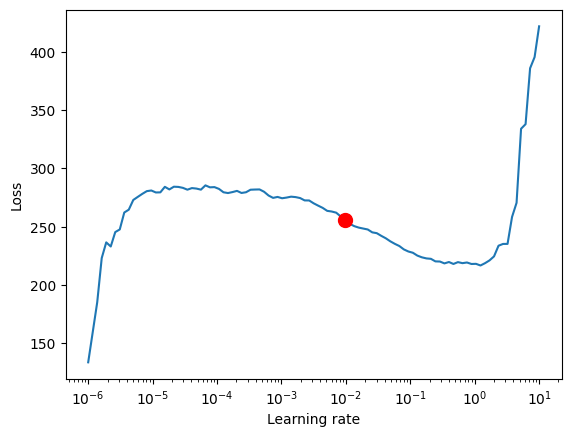For the TemporalFusionTransformer, the optimal learning rate seems to be slightly lower than the suggested one. Further, we do not directly want to use the suggested learning rate because PyTorch Lightning sometimes can get confused by the noise at lower learning rates and suggests rates far too low. Manual control is essential. We decide to pick 0.03 as learning rate.

### Train model#

If you have troubles training the model and get an error AttributeError: module 'tensorflow._api.v2.io.gfile' has no attribute 'get_filesystem', consider either uninstalling tensorflow or first execute

import tensorflow as tf
import tensorboard as tb
tf.io.gfile = tb.compat.tensorflow_stub.io.gfile
.

:

# configure network and trainer
early_stop_callback = EarlyStopping(monitor="val_loss", min_delta=1e-4, patience=10, verbose=False, mode="min")
lr_logger = LearningRateMonitor()  # log the learning rate
logger = TensorBoardLogger("lightning_logs")  # logging results to a tensorboard

trainer = pl.Trainer(
max_epochs=50,
accelerator="cpu",
enable_model_summary=True,
limit_train_batches=50,  # coment in for training, running valiation every 30 batches
# fast_dev_run=True,  # comment in to check that networkor dataset has no serious bugs
callbacks=[lr_logger, early_stop_callback],
logger=logger,
)

tft = TemporalFusionTransformer.from_dataset(
training,
learning_rate=0.03,
hidden_size=16,
dropout=0.1,
hidden_continuous_size=8,
loss=QuantileLoss(),
log_interval=10,  # uncomment for learning rate finder and otherwise, e.g. to 10 for logging every 10 batches
optimizer="Ranger",
reduce_on_plateau_patience=4,
)
print(f"Number of parameters in network: {tft.size()/1e3:.1f}k")

GPU available: True (mps), used: False
TPU available: False, using: 0 TPU cores
IPU available: False, using: 0 IPUs
HPU available: False, using: 0 HPUs

Number of parameters in network: 29.4k


Training takes a couple of minutes on my Macbook but for larger networks and datasets, it can take hours. The training speed is here mostly determined by overhead and choosing a larger batch_size or hidden_size (i.e. network size) does not slow does training linearly making training on large datasets feasible. During training, we can monitor the tensorboard which can be spun up with tensorboard --logdir=lightning_logs. For example, we can monitor examples predictions on the training and validation set.

:

# fit network
trainer.fit(
tft,
)


| Name                               | Type                            | Params
----------------------------------------------------------------------------------------
0  | loss                               | QuantileLoss                    | 0
1  | logging_metrics                    | ModuleList                      | 0
2  | input_embeddings                   | MultiEmbedding                  | 1.3 K
3  | prescalers                         | ModuleDict                      | 256
4  | static_variable_selection          | VariableSelectionNetwork        | 3.4 K
5  | encoder_variable_selection         | VariableSelectionNetwork        | 8.0 K
6  | decoder_variable_selection         | VariableSelectionNetwork        | 2.7 K
7  | static_context_variable_selection  | GatedResidualNetwork            | 1.1 K
8  | static_context_initial_hidden_lstm | GatedResidualNetwork            | 1.1 K
9  | static_context_initial_cell_lstm   | GatedResidualNetwork            | 1.1 K
10 | static_context_enrichment          | GatedResidualNetwork            | 1.1 K
11 | lstm_encoder                       | LSTM                            | 2.2 K
12 | lstm_decoder                       | LSTM                            | 2.2 K
13 | post_lstm_gate_encoder             | GatedLinearUnit                 | 544
15 | static_enrichment                  | GatedResidualNetwork            | 1.4 K
17 | post_attn_gate_norm                | GateAddNorm                     | 576
18 | pos_wise_ff                        | GatedResidualNetwork            | 1.1 K
19 | pre_output_gate_norm               | GateAddNorm                     | 576
20 | output_layer                       | Linear                          | 119
----------------------------------------------------------------------------------------
29.4 K    Trainable params
0         Non-trainable params
29.4 K    Total params
0.118     Total estimated model params size (MB)


### Hyperparameter tuning#

Hyperparamter tuning with [optuna](https://optuna.org/) is directly build into pytorch-forecasting. For example, we can use the optimize_hyperparameters() function to optimize the TFT’s hyperparameters.

import pickle

from pytorch_forecasting.models.temporal_fusion_transformer.tuning import optimize_hyperparameters

# create study
study = optimize_hyperparameters(
model_path="optuna_test",
n_trials=200,
max_epochs=50,
hidden_size_range=(8, 128),
hidden_continuous_size_range=(8, 128),
learning_rate_range=(0.001, 0.1),
dropout_range=(0.1, 0.3),
trainer_kwargs=dict(limit_train_batches=30),
reduce_on_plateau_patience=4,
use_learning_rate_finder=False,  # use Optuna to find ideal learning rate or use in-built learning rate finder
)

# save study results - also we can resume tuning at a later point in time
with open("test_study.pkl", "wb") as fout:
pickle.dump(study, fout)

# show best hyperparameters
print(study.best_trial.params)


## Evaluate performance#

PyTorch Lightning automatically checkpoints training and thus, we can easily retrieve the best model and load it.

:

# load the best model according to the validation loss
# (given that we use early stopping, this is not necessarily the last epoch)
best_model_path = trainer.checkpoint_callback.best_model_path


After training, we can make predictions with predict(). The method allows very fine-grained control over what it returns so that, for example, you can easily match predictions to your pandas dataframe. See its documentation for details. We evaluate the metrics on the validation dataset and a couple of examples to see how well the model is doing. Given that we work with only 21 000 samples the results are very reassuring and can compete with results by a gradient booster. We also perform better than the baseline model. Given the noisy data, this is not trivial.

:

# calcualte mean absolute error on validation set
MAE()(predictions.output, predictions.y)

:

tensor(262.1610)


We can now also look at sample predictions directly which we plot with plot_prediction(). As you can see from the figures below, forecasts look rather accurate. If you wonder, the grey lines denote the amount of attention the model pays to different points in time when making the prediction. This is a special feature of the Temporal Fusion Transformer.

:

# raw predictions are a dictionary from which all kind of information including quantiles can be extracted

:

for idx in range(10):  # plot 10 examples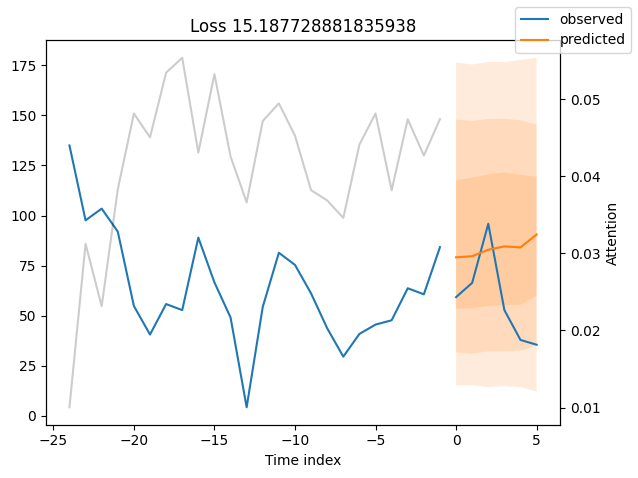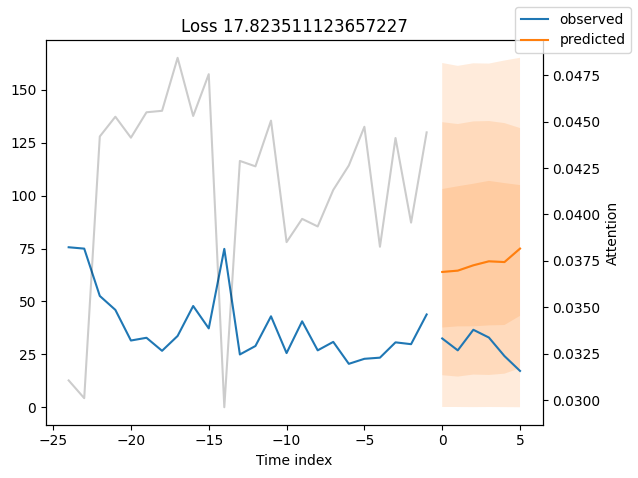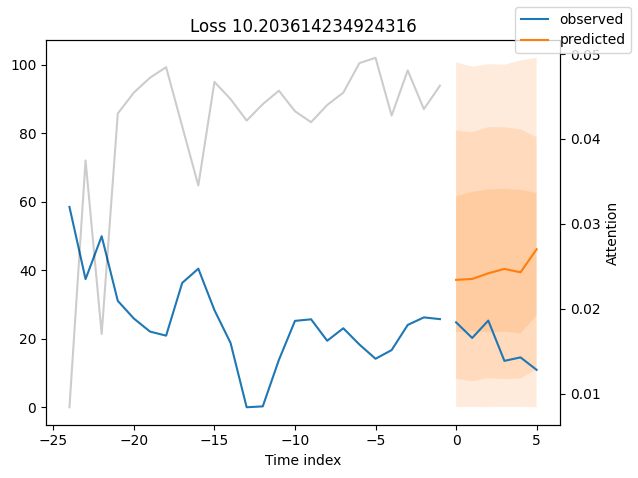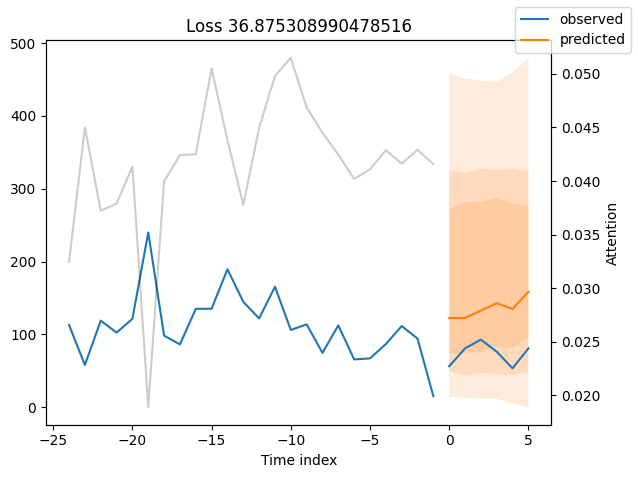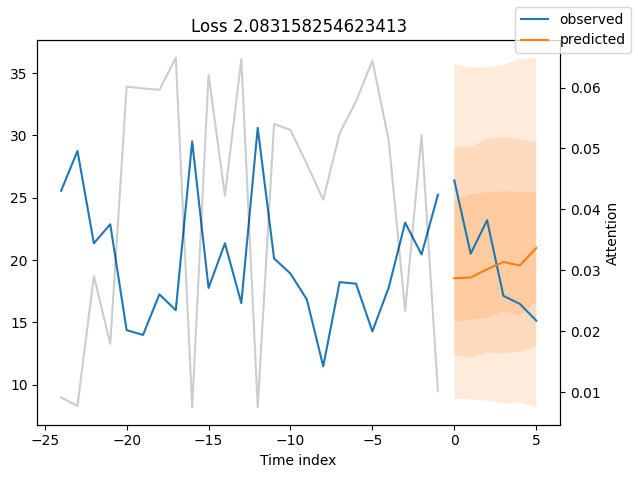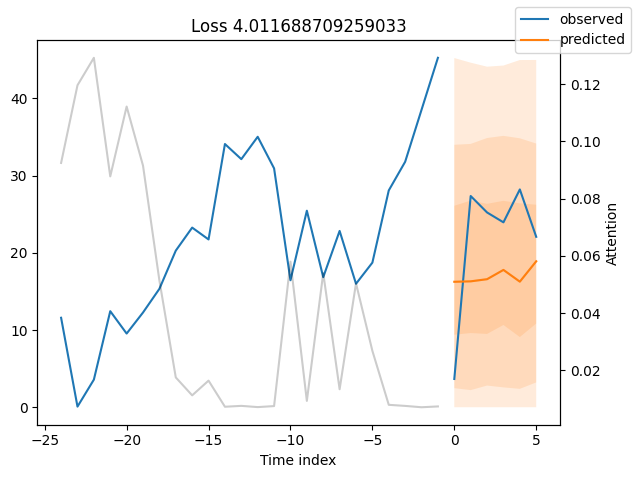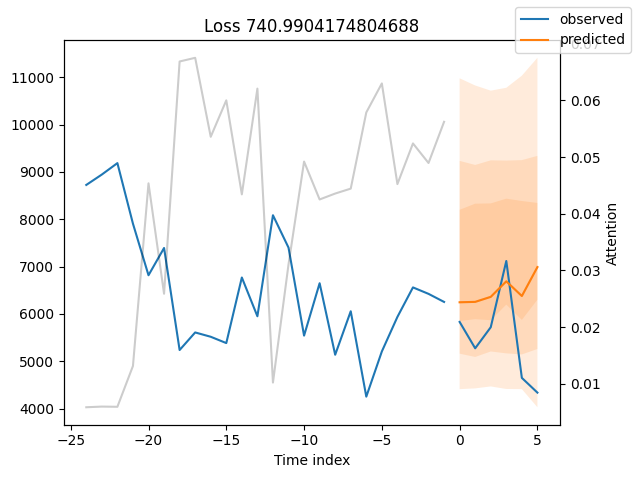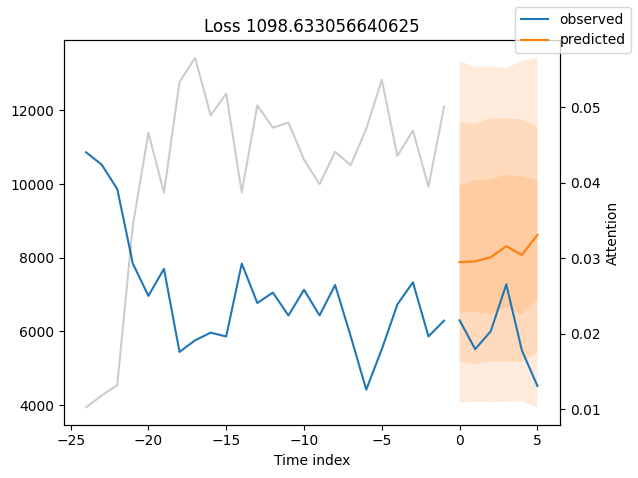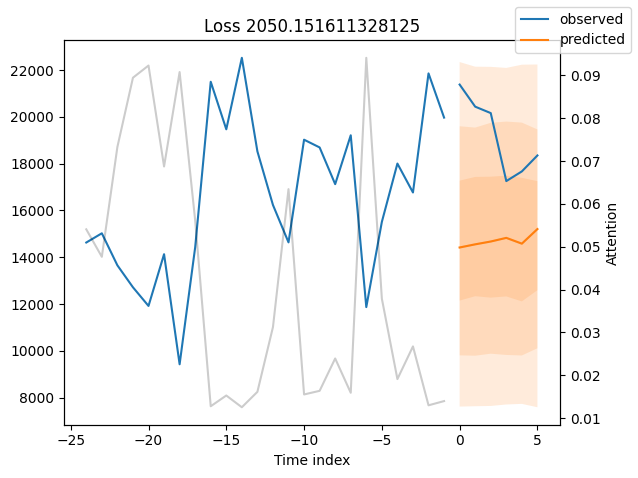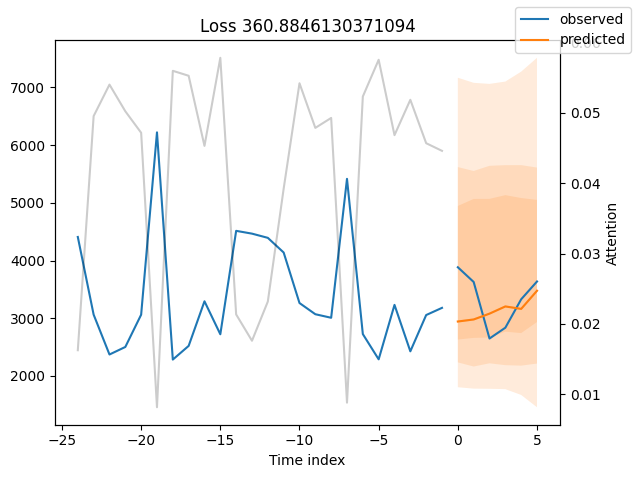### Worst performers#

Looking at the worst performers, for example in terms of SMAPE, gives us an idea where the model has issues with forecasting reliably. These examples can provide important pointers about how to improve the model. This kind of actuals vs predictions plots are available to all models. Of course, it is also sensible to employ additional metrics, such as MASE, defined in the metrics module. However, for the sake of demonstration, we only use SMAPE here.

:

# calcualte metric by which to display
mean_losses = SMAPE(reduction="none")(predictions.output, predictions.y).mean(1)
indices = mean_losses.argsort(descending=True)  # sort losses
for idx in range(10):  # plot 10 examples
best_tft.plot_prediction(
raw_predictions.x,
raw_predictions.output,
idx=indices[idx],
)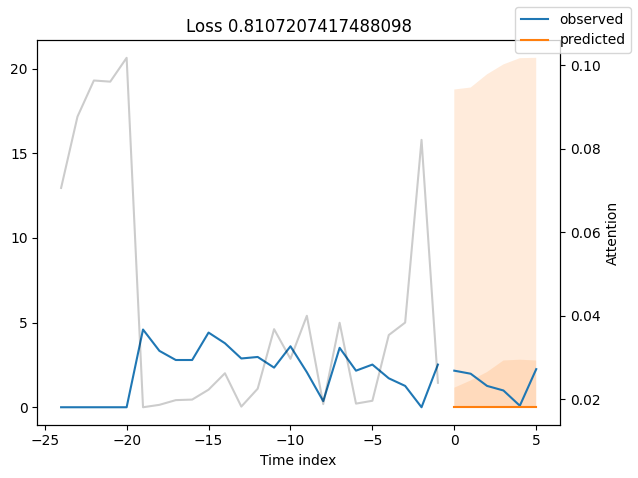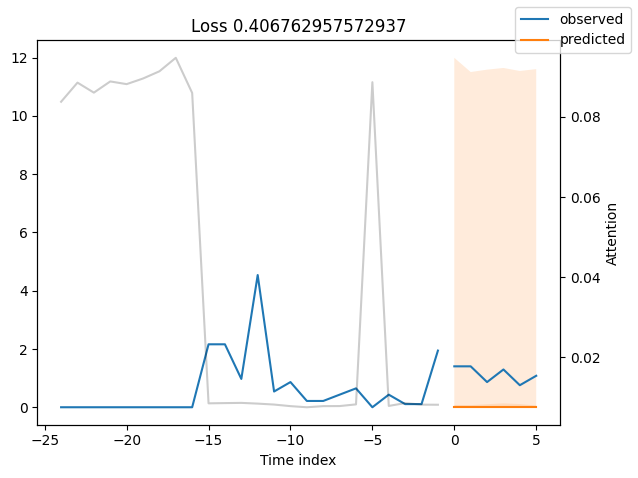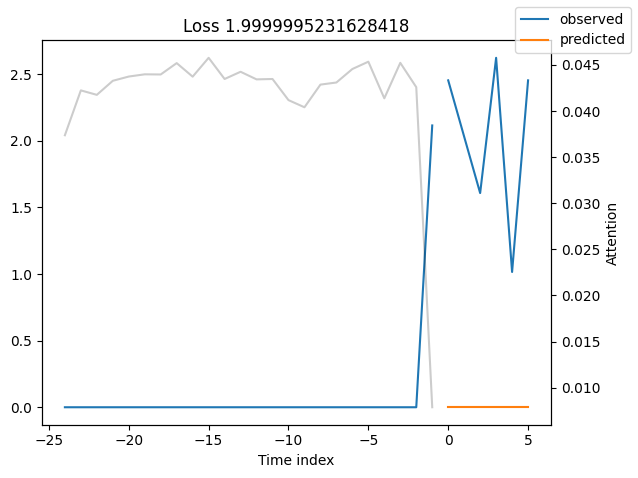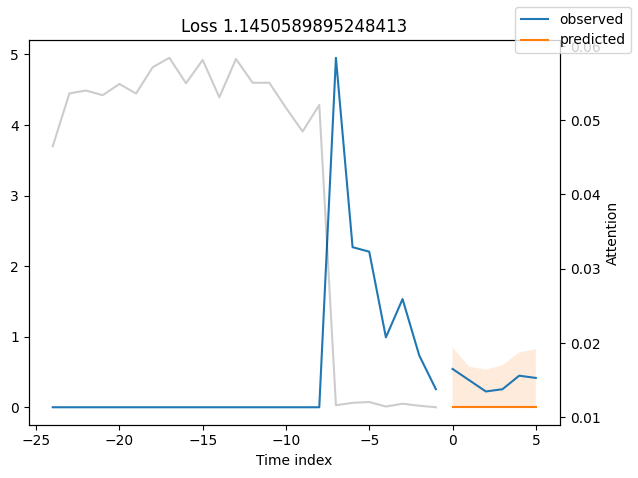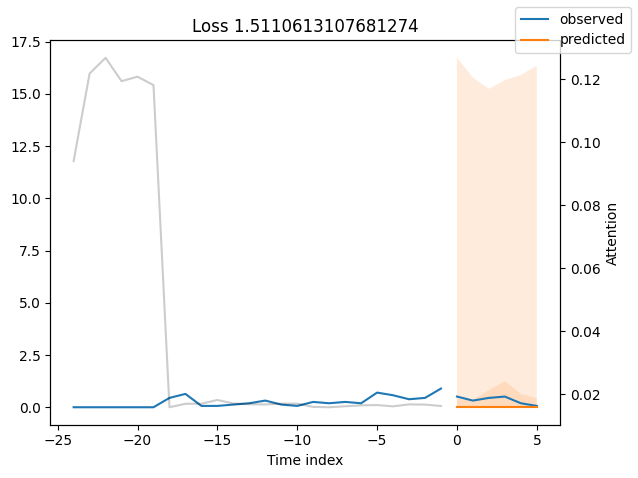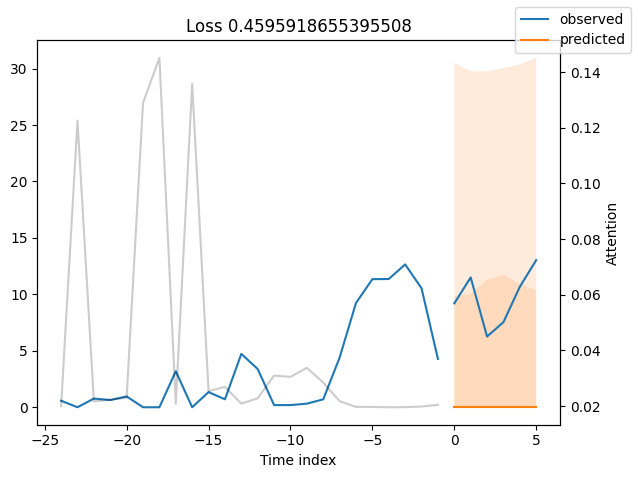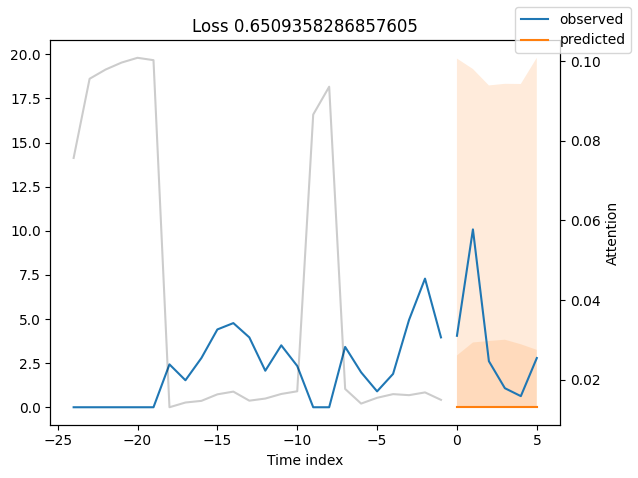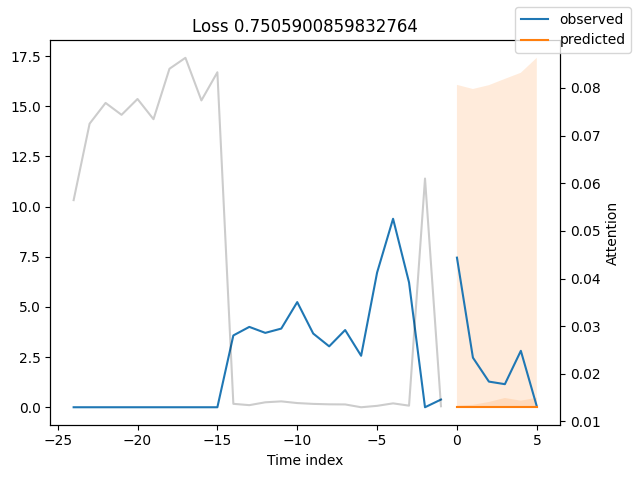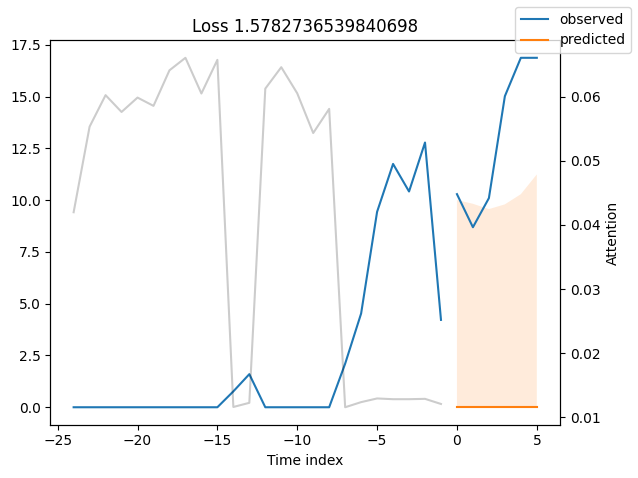### Actuals vs predictions by variables#

Checking how the model performs across different slices of the data allows us to detect weaknesses. Plotted below are the means of predictions vs actuals across each variable divided into 100 bins using the Now, we can directly predict on the generated data using the calculate_prediction_actual_by_variable() and plot_prediction_actual_by_variable() methods. The gray bars denote the frequency of the variable by bin, i.e. are a histogram.

:

predictions = best_tft.predict(val_dataloader, return_x=True)
predictions_vs_actuals = best_tft.calculate_prediction_actual_by_variable(predictions.x, predictions.output)
best_tft.plot_prediction_actual_by_variable(predictions_vs_actuals)

:

{'avg_population_2017': <Figure size 1000x500 with 2 Axes>,
'avg_yearly_household_income_2017': <Figure size 1000x500 with 2 Axes>,
'encoder_length': <Figure size 1000x500 with 2 Axes>,
'volume_center': <Figure size 1000x500 with 2 Axes>,
'volume_scale': <Figure size 1000x500 with 2 Axes>,
'time_idx': <Figure size 1000x500 with 2 Axes>,
'price_regular': <Figure size 1000x500 with 2 Axes>,
'discount_in_percent': <Figure size 1000x500 with 2 Axes>,
'relative_time_idx': <Figure size 1000x500 with 2 Axes>,
'volume': <Figure size 1000x500 with 2 Axes>,
'log_volume': <Figure size 1000x500 with 2 Axes>,
'industry_volume': <Figure size 1000x500 with 2 Axes>,
'soda_volume': <Figure size 1000x500 with 2 Axes>,
'avg_max_temp': <Figure size 1000x500 with 2 Axes>,
'avg_volume_by_agency': <Figure size 1000x500 with 2 Axes>,
'avg_volume_by_sku': <Figure size 1000x500 with 2 Axes>,
'agency': <Figure size 1000x500 with 2 Axes>,
'sku': <Figure size 1000x500 with 2 Axes>,
'special_days': <Figure size 1000x500 with 2 Axes>,
'month': <Figure size 1000x500 with 2 Axes>}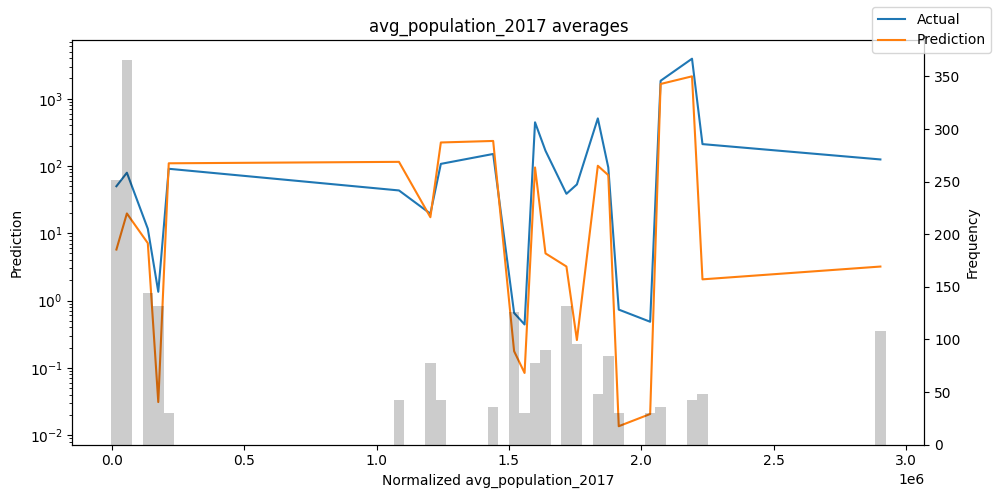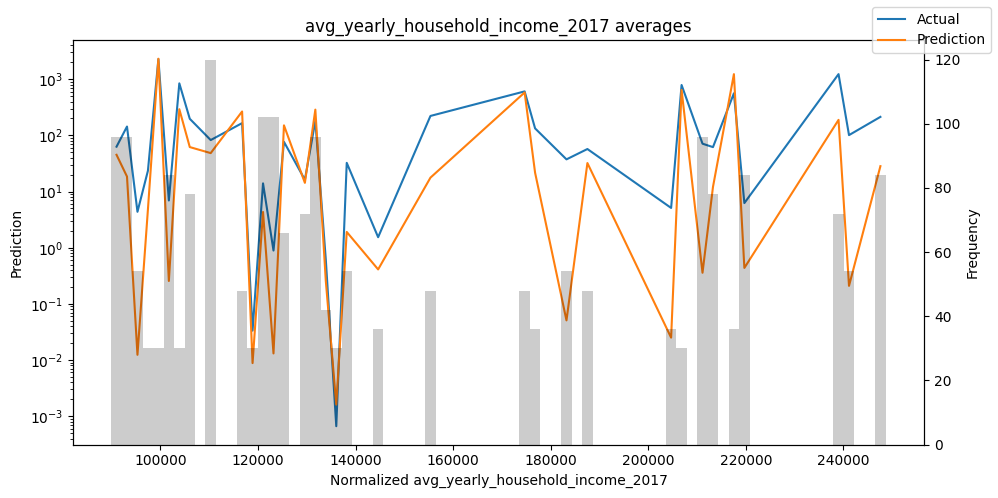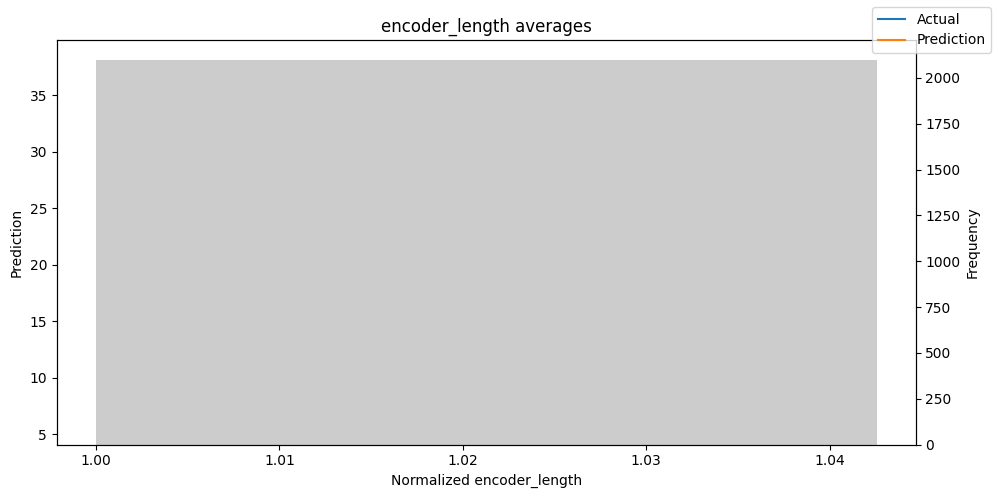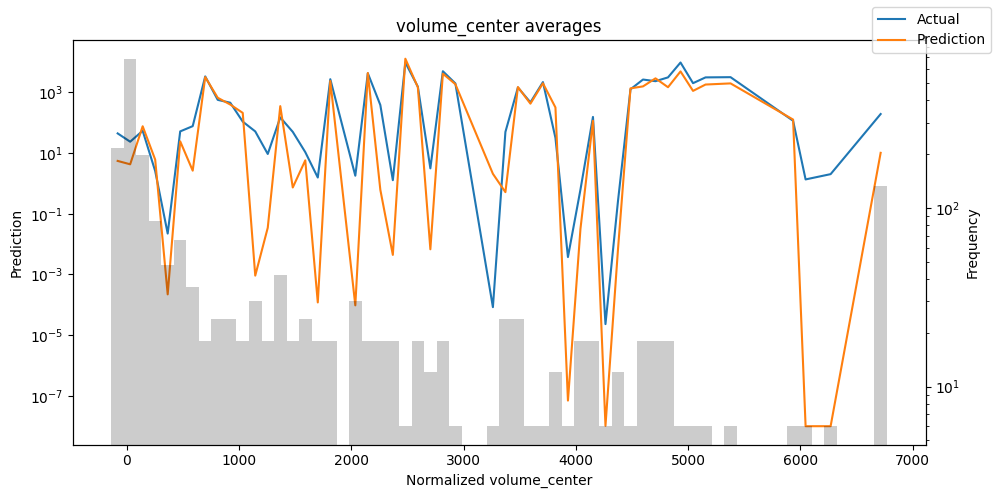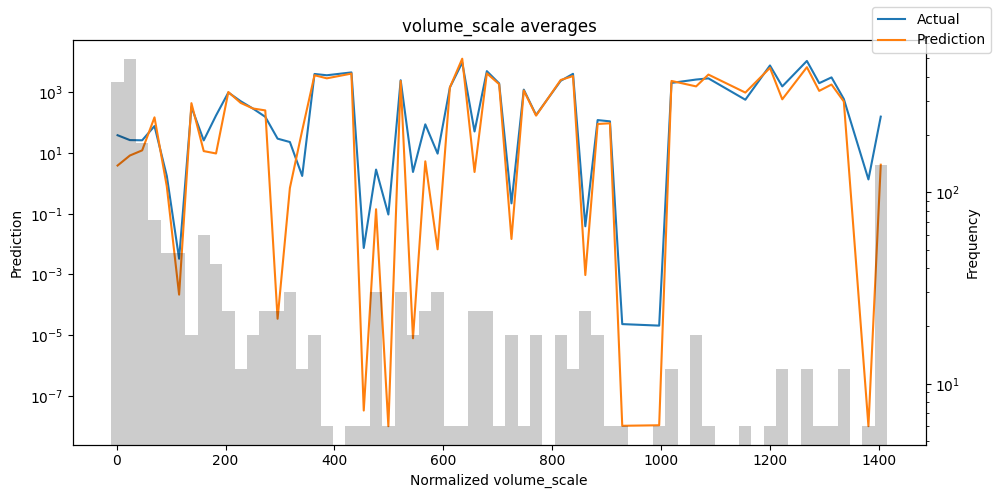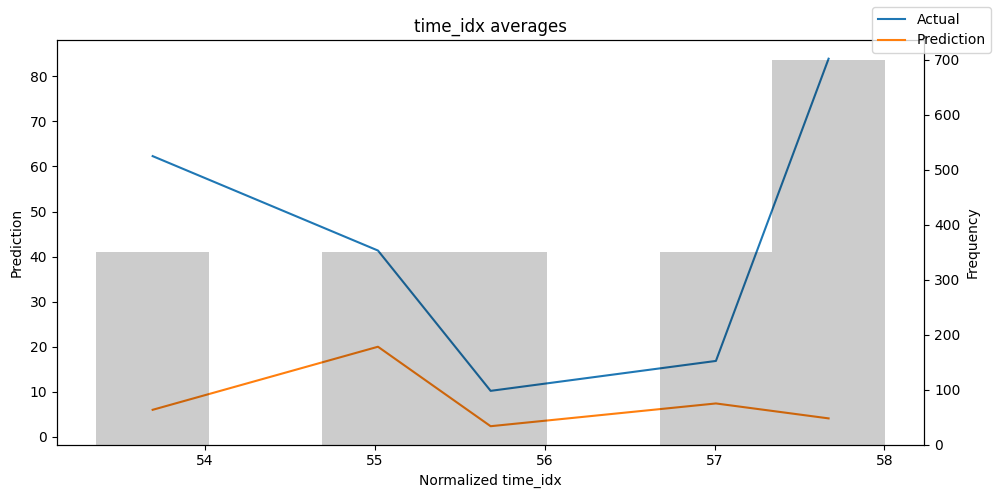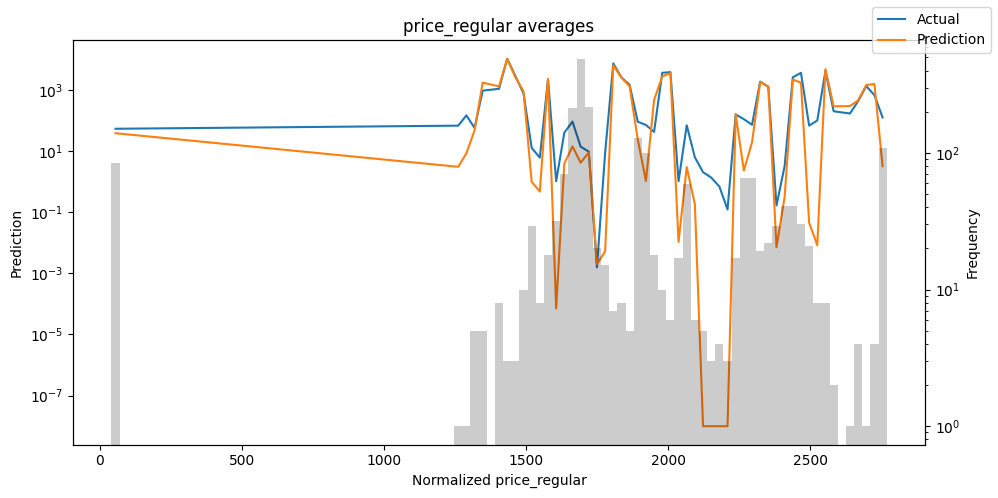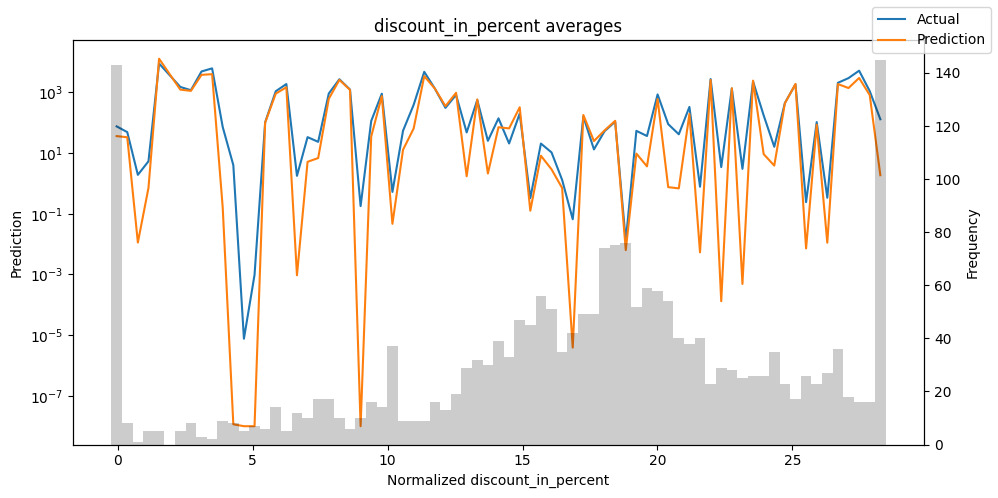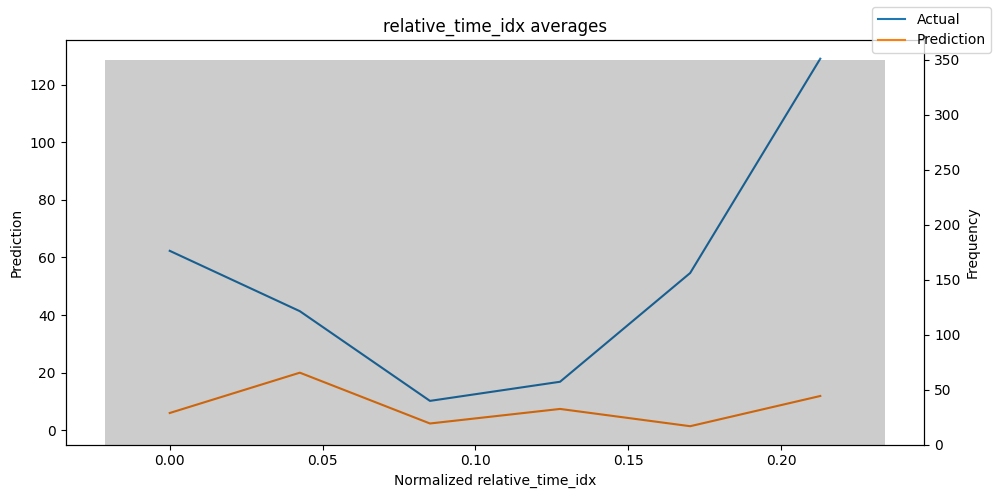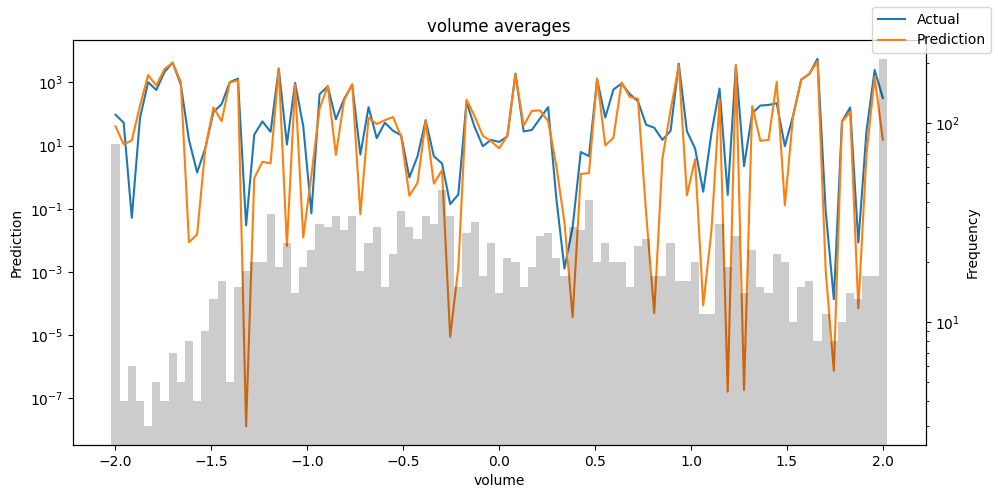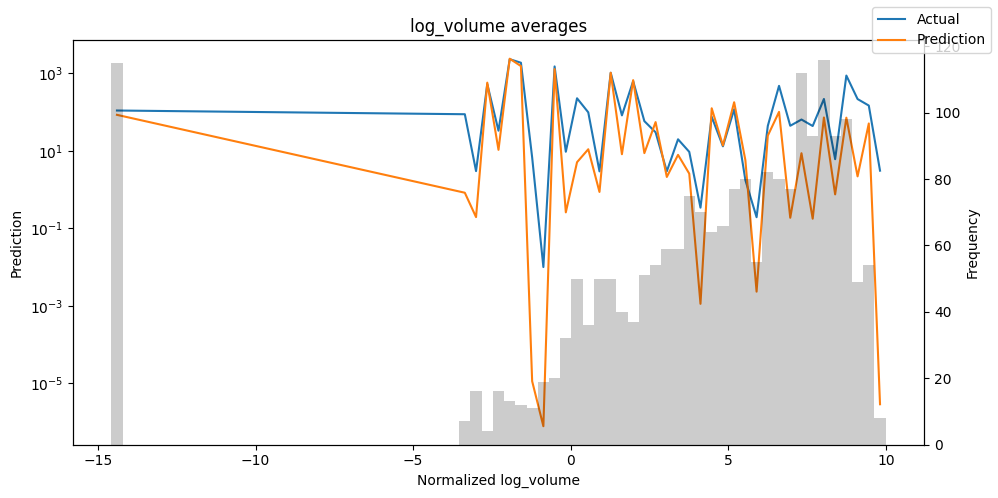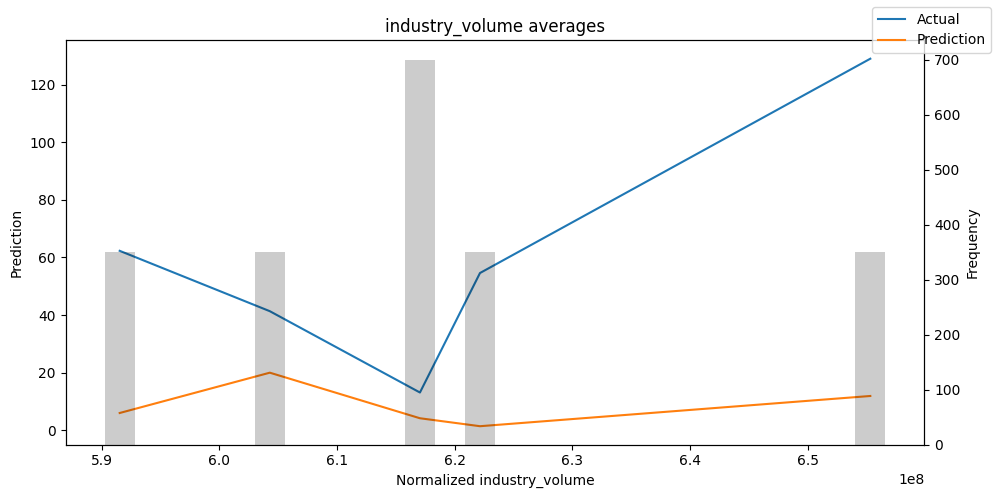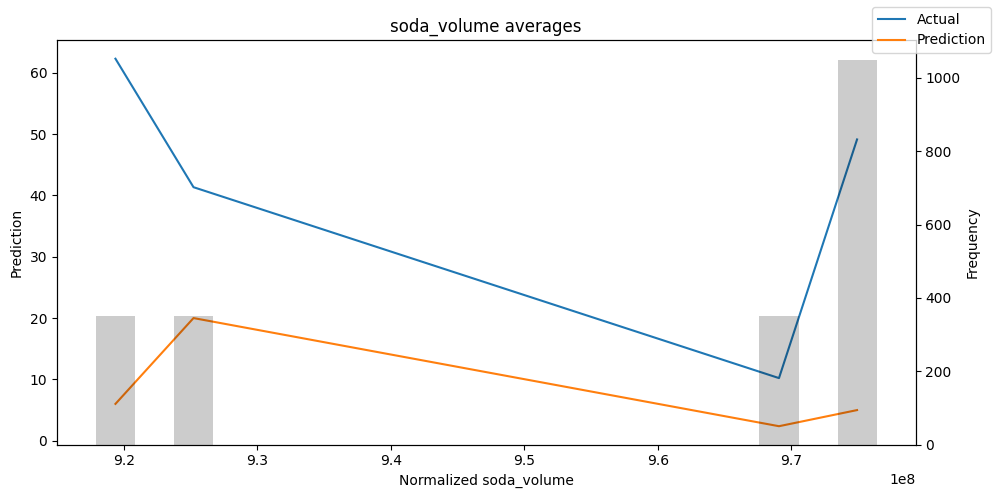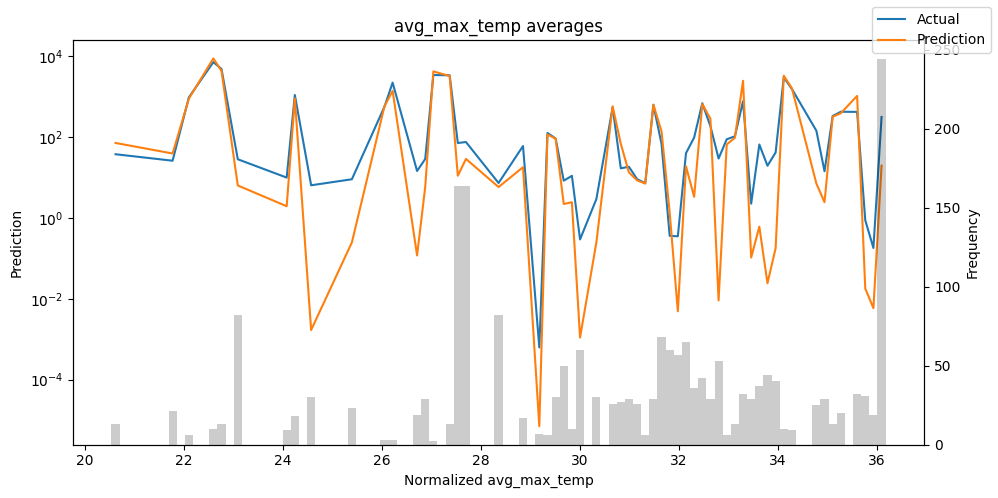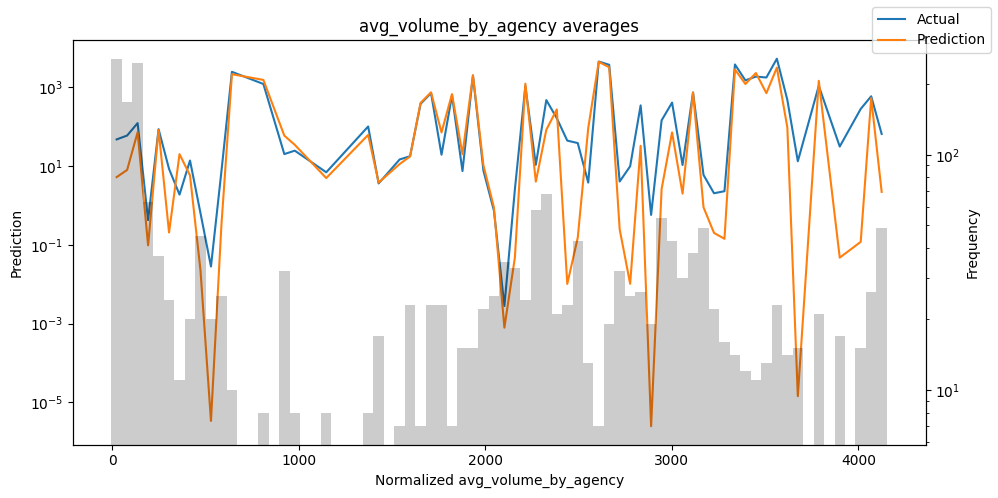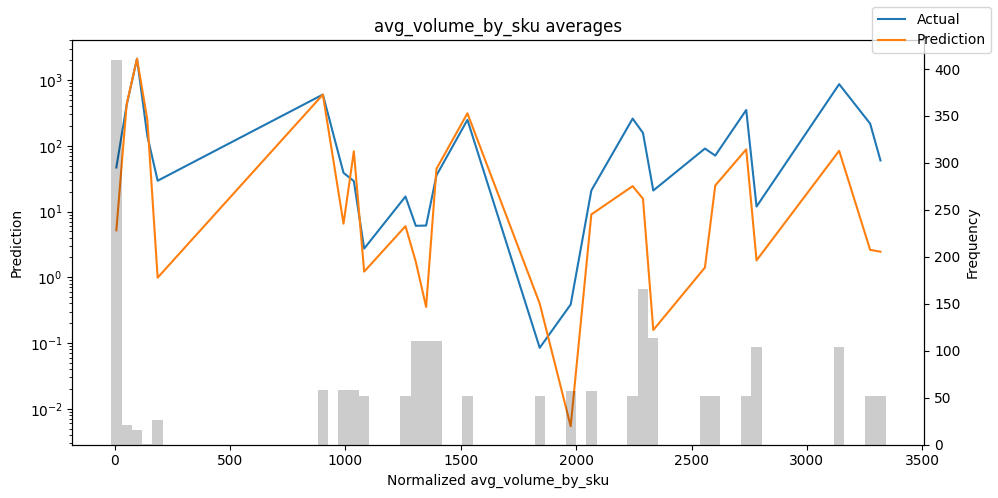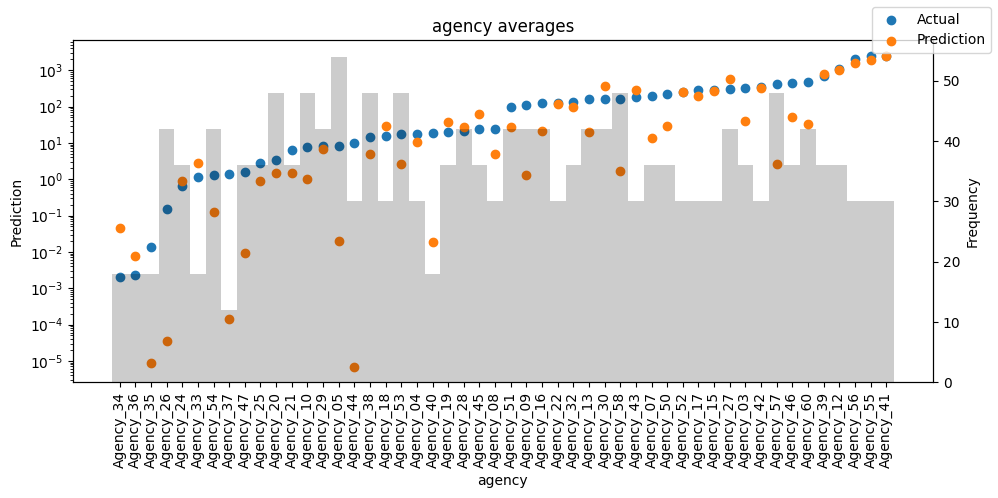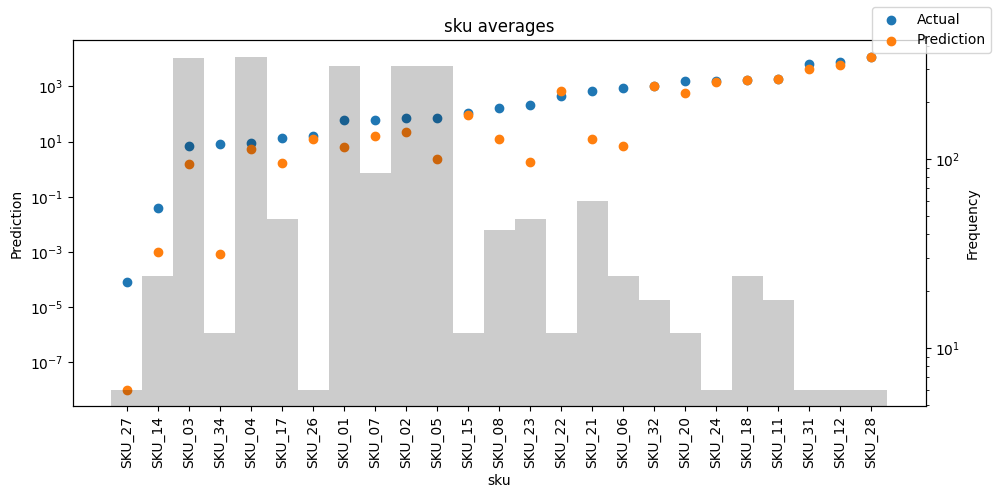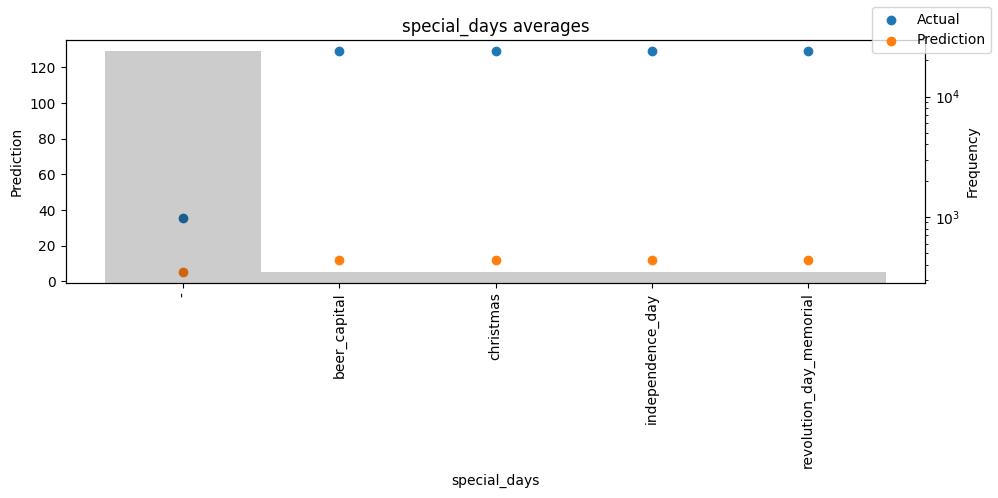## Predict on selected data#

To predict on a subset of data we can filter the subsequences in a dataset using the filter() method. Here we predict for the subsequence in the training dataset that maps to the group ids “Agency_01” and “SKU_01” and whose first predicted value corresponds to the time index “15”. We output all seven quantiles. This means we expect a tensor of shape 1 x n_timesteps x n_quantiles = 1 x 6 x 7 as we predict for a single subsequence six time steps ahead and 7 quantiles for each time step.

:

best_tft.predict(
training.filter(lambda x: (x.agency == "Agency_01") & (x.sku == "SKU_01") & (x.time_idx_first_prediction == 15)),
mode="quantiles",
)

:

tensor([[[ 71.8401,  95.7425, 110.2563, 130.6213, 147.2689, 164.7592, 183.4534],
[ 26.5545,  56.0831,  73.5964,  98.4364, 117.8405, 141.8476, 163.2200],
[ 19.5155,  46.8377,  62.4305,  86.6114, 104.1645, 126.8418, 147.6066],
[ 34.3064,  58.6321,  72.5809,  93.5944, 110.5706, 129.0367, 154.7733],
[ 28.2280,  53.3502,  67.4921,  88.0191, 104.0002, 120.7509, 141.0733],
[ 17.6741,  42.3217,  55.3259,  77.8475,  93.1427, 112.8938, 135.0351]]],
device='mps:0')


Of course, we can also plot this prediction readily:

:

raw_prediction = best_tft.predict(
training.filter(lambda x: (x.agency == "Agency_01") & (x.sku == "SKU_01") & (x.time_idx_first_prediction == 15)),
mode="raw",
return_x=True,
)
best_tft.plot_prediction(raw_prediction.x, raw_prediction.output, idx=0)

: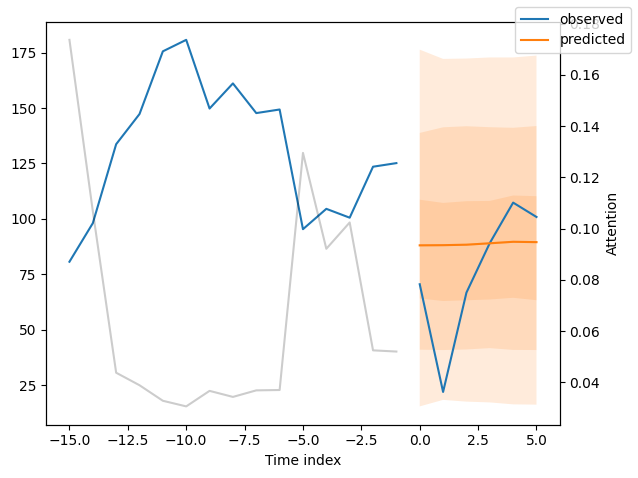## Predict on new data#

Because we have covariates in the dataset, predicting on new data requires us to define the known covariates upfront.

:

# select last 24 months from data (max_encoder_length is 24)
encoder_data = data[lambda x: x.time_idx > x.time_idx.max() - max_encoder_length]

# select last known data point and create decoder data from it by repeating it and incrementing the month
# in a real world dataset, we should not just forward fill the covariates but specify them to account
# for changes in special days and prices (which you absolutely should do but we are too lazy here)
last_data = data[lambda x: x.time_idx == x.time_idx.max()]
decoder_data = pd.concat(
[last_data.assign(date=lambda x: x.date + pd.offsets.MonthBegin(i)) for i in range(1, max_prediction_length + 1)],
ignore_index=True,
)

# add time index consistent with "data"
decoder_data["time_idx"] = decoder_data["date"].dt.year * 12 + decoder_data["date"].dt.month
decoder_data["time_idx"] += encoder_data["time_idx"].max() + 1 - decoder_data["time_idx"].min()

decoder_data["month"] = decoder_data.date.dt.month.astype(str).astype("category")  # categories have be strings

# combine encoder and decoder data
new_prediction_data = pd.concat([encoder_data, decoder_data], ignore_index=True)


Now, we can directly predict on the generated data using the predict() method.

:

new_raw_predictions = best_tft.predict(new_prediction_data, mode="raw", return_x=True)

for idx in range(10):  # plot 10 examples
best_tft.plot_prediction(new_raw_predictions.x, new_raw_predictions.output, idx=idx, show_future_observed=False)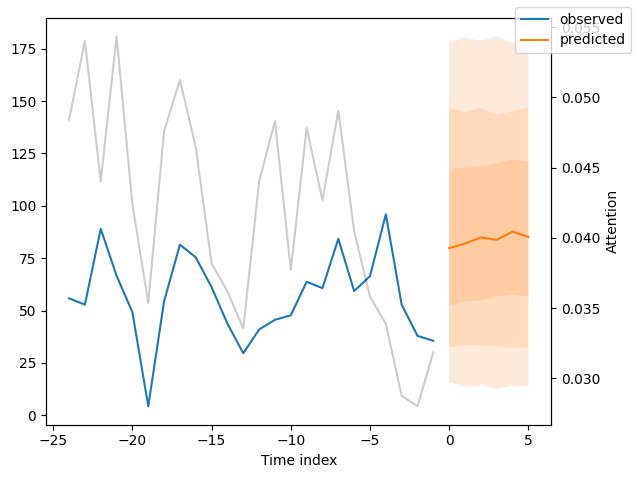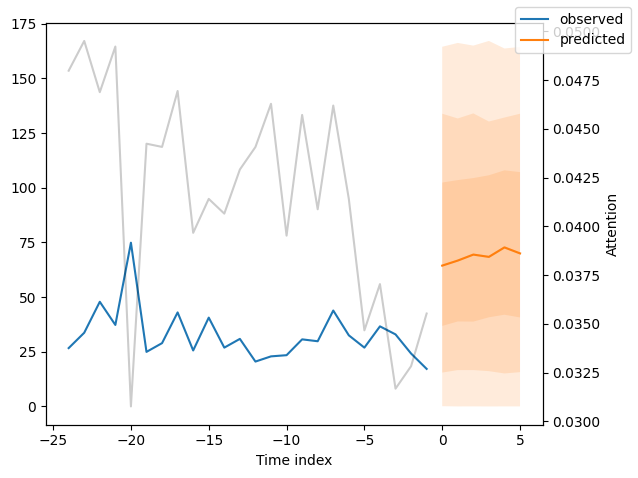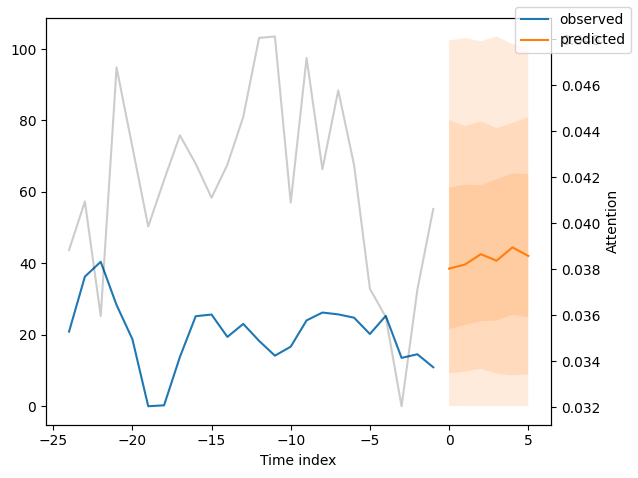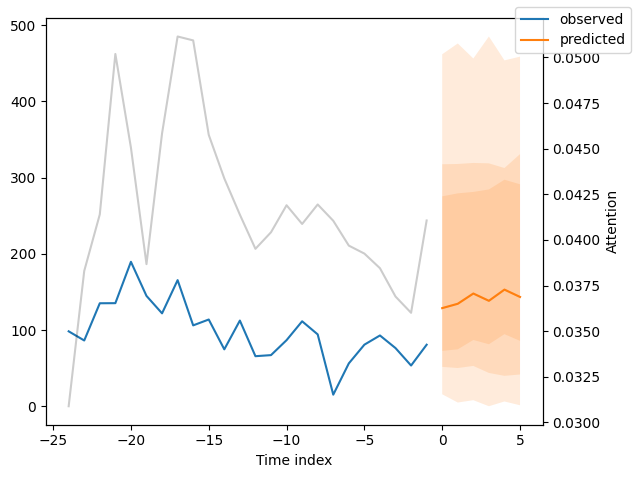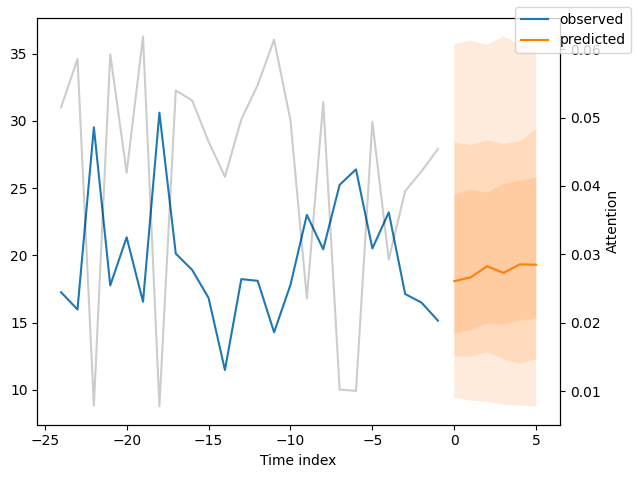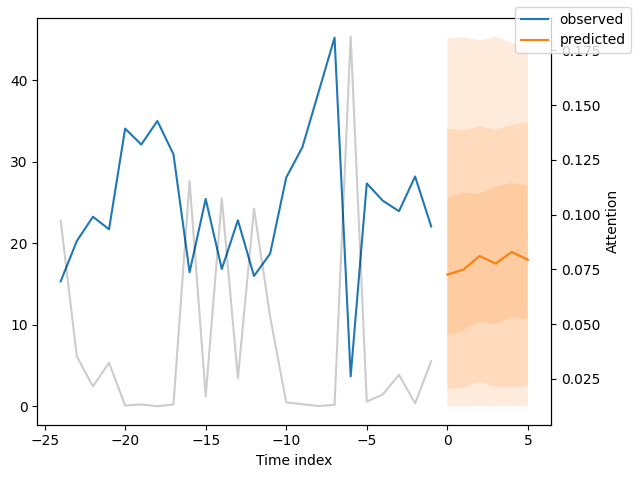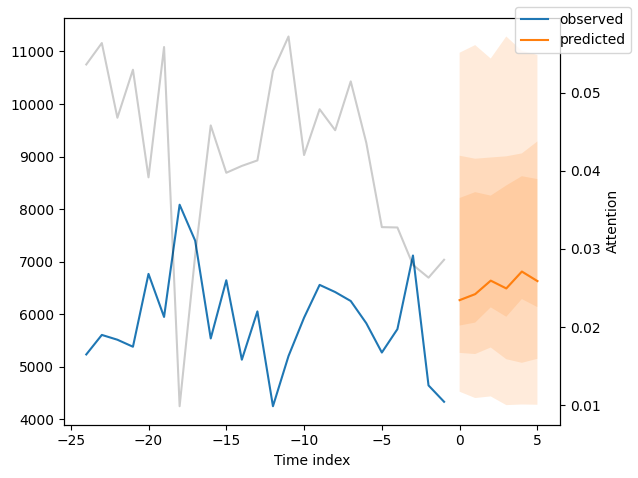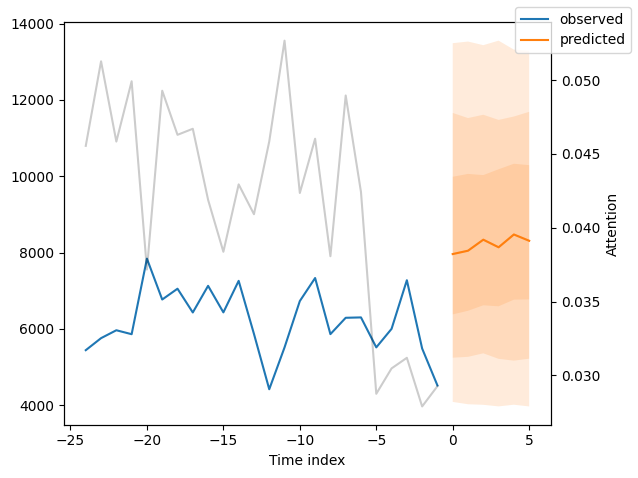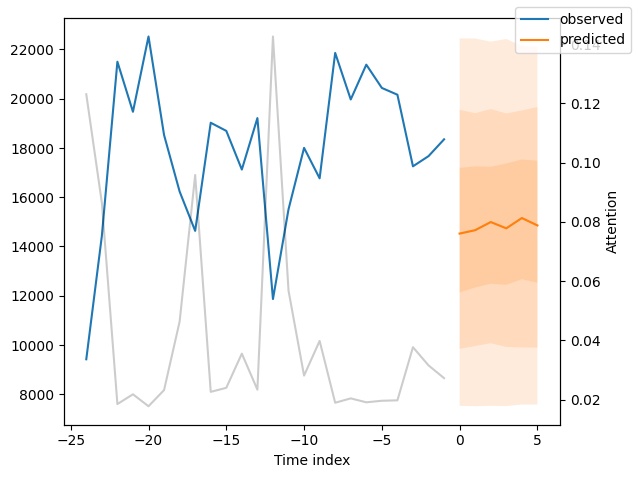## Interpret model#

### Variable importances#

The model has inbuilt interpretation capabilities due to how its architecture is build. Let’s see how that looks. We first calculate interpretations with interpret_output() and plot them subsequently with plot_interpretation().

:

interpretation = best_tft.interpret_output(raw_predictions.output, reduction="sum")
best_tft.plot_interpretation(interpretation)

:

{'attention': <Figure size 640x480 with 1 Axes>,
'static_variables': <Figure size 700x375 with 1 Axes>,
'encoder_variables': <Figure size 700x525 with 1 Axes>,
'decoder_variables': <Figure size 700x350 with 1 Axes>}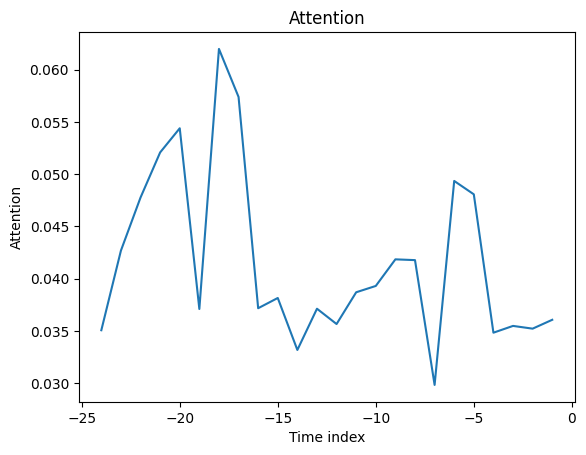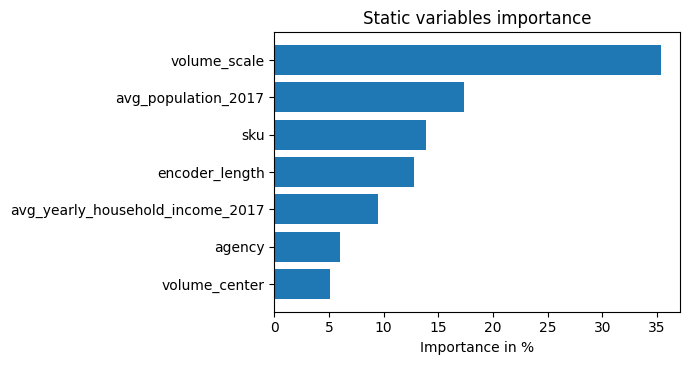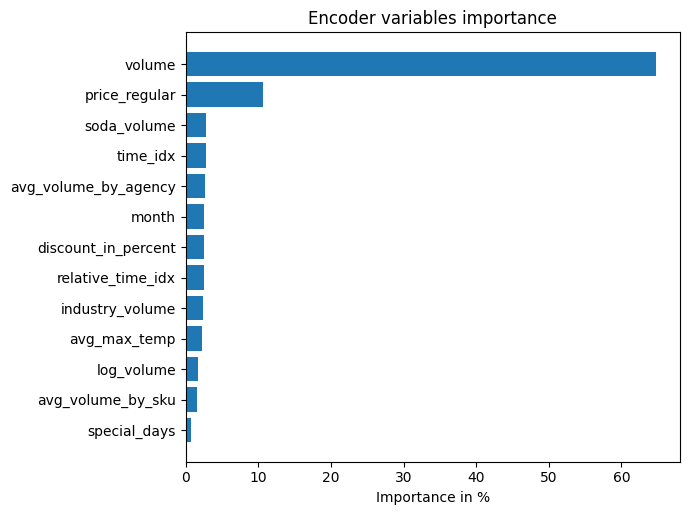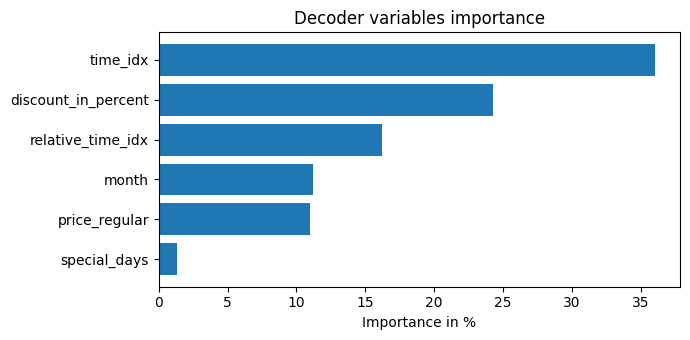Unsurprisingly, the past observed volume features as the top variable in the encoder and price related variables are among the top predictors in the decoder.

The general attention patterns seems to be that more recent observations are more important and older ones. This confirms intuition. The average attention is often not very useful - looking at the attention by example is more insightful because patterns are not averaged out.

### Partial dependency#

Partial dependency plots are often used to interpret the model better (assuming independence of features). They can be also useful to understand what to expect in case of simulations and are created with predict_dependency().

:

dependency = best_tft.predict_dependency(
val_dataloader.dataset, "discount_in_percent", np.linspace(0, 30, 30), show_progress_bar=True, mode="dataframe"
)

:

# plotting median and 25% and 75% percentile
agg_dependency = dependency.groupby("discount_in_percent").normalized_prediction.agg(
median="median", q25=lambda x: x.quantile(0.25), q75=lambda x: x.quantile(0.75)
)
ax = agg_dependency.plot(y="median")
ax.fill_between(agg_dependency.index, agg_dependency.q25, agg_dependency.q75, alpha=0.3)

:

<matplotlib.collections.PolyCollection at 0x2db63e800>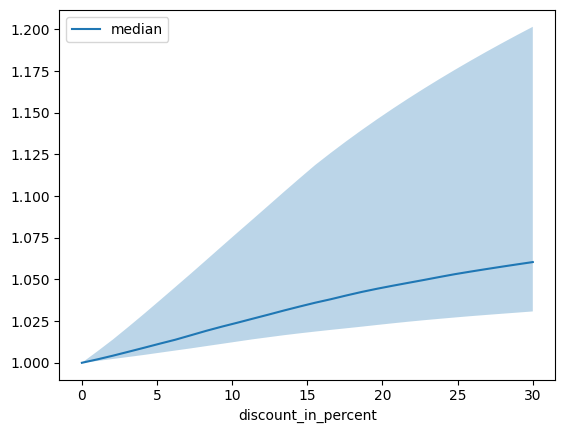[ ]:


`